Time: 3 Hours                                                                                                     Max. Marks: 100

NOTE: There are 9 Questions in all.

·      Question 1 is compulsory and carries 20 marks. Answer to Q. 1. must be written in the space provided for it in the answer book supplied and nowhere else.

·      Out of the remaining EIGHT Questions answer any FIVE Questions. Each question carries 16 marks.

·      Any required data not explicitly given, may be suitably assumed and stated.

Q.1       Choose the correct or best alternative in the following:                                         (2x10)

a.       Which of the following are ways to specify the position of a point on the screen?

(A)    Relative co-ordinates                    (B)  absolute co-ordinates

(C) both (A) and (B)                           (D)  none of the above

b.      If the height of image is 8 inch and the aspect ratio is ¾, what is the width of the image?

(A) 4 inches                                         (B)  6 inches

(C) 8 inches                                         (D)  none of the above

c.   For the line joining (3, 5) and (15, 6) the location of the second pixel is at

(A)    (3, 6)                                           (B)  (4, 5)

(C) (4, 6)                                            (D)  none of the above

d.   Which of the following data structures is used for seed fill boundary-defined regions?

(A)   tree                                              (B)  queue

e.   The 3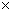3 pattern, in half toning is given below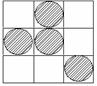Can be represented by the matrix

(A)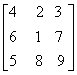(B)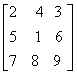(C)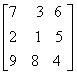(D)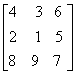f.    The AND operation of the codes of the end points of a line completely outside the clip window

(A)     may be equal to (0000)                (B)  will never be equal to (0000)

(C)  shall be nonzero always                (D)  none of the above

g.   Bezier curves with control points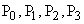and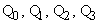are joined at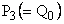, for first order continuity at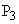we must have

(A)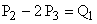(B)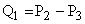(C)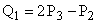(D)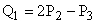h.   The following knot vector

[0, 0, 0, 0, 1 , 2, 2, 2, 2] represents

(A)    Bezier spline                                 (B) open uniform B-spline

(C) Periodic B-spline                           (D) Non-uniform B-spline

i.    If a quadtree has 4 levels then the area represent by it has at most_____pixels.

(A)   16                                                (B) 64

(C) 128                                               (D) 256

j.    Given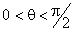, to model increasing speed in an animator we use

(A)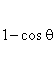(B)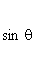(C)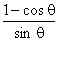(D)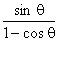Answer any FIVE Questions out of EIGHT Questions.

Each question carries 16 marks.

Q.2     a.   Describe the architecture of a raster graphic system with display precessor, explaining the tasks performed by display processor.                           (8)

b.   Using Bresenham’s algorithm give the location of the pixels for the line joining the points (-1, -1) and (-9, -5)                                                           (8)

Q.3     a.   Derive the transformation matrix in 2-D for the shear transformation relative to line x = 10.              (5)

b.   Using seed fill algorithm for 4-connected regions give the locations of first five pixels. The seed is the location (8,9) and the polygon is with the vertices (5, 12), (20,12) (20,10), (10,10), (10, 6) and (5,6).        (8)

c.   Give the advantages of Cyrus-Beck algorithm over Cohen Sutherland algorithm.                 (3)

Q.4     a.   Show that two successive scaling operations are multiplicative.                            (3)

b.   Give the composite transformation matrix of an object in 3D if the subject is scaled, rotated about Y-axis and then translated.                                    (6)

c.   Find perspective projection of point (-1, -2, -3) onto the plane z = 6 when the projection reference point is (0, 0, -8).                                            (7)

Q.5     a.   ‘Bezier Curve do not allow for local control of the curve shape’. Justify.              (6)

b.   Give the blending functions for uniform quadratic B-spline with knot vectors [0, 1, 2, 3, 4, 5, 6]                                                                 (6)

c.   What are the boundary conditions for periodic cubic splines with four control points?                      (4)

Q.6   a.    Write the octree display algorithm for solids.                                                      (4)

b.   What do you mean by binary space partioning tree? Draw a binary space partioning tree for a triangle.                                                                   (6)

c.   What do you mean by intensity attenuation? Give the reasons for tasking inverse quadratic function as attenuation function.                             (6)

Q.7     a.   What do you mean by self similar fractals? Give an example.                               (4)

b.   How are complex number mapping used for generating fractals? Explain.            (8)

c.   Briefly explain sweep representations for modelling 3-D object.           (4)

Q.8     a.   Describe Phong shading model. How is it more accurate than Gourand shading model?                   (8)

b.   Write Painter’s algorithm for detecting hidden surfaces.                                       (8)

Q.9     a.   Describe combined diffuse and specular reflection model including attenuation factor with multiple light sources.                                               (6)

b.   Differentiate between parallel and perspective projections.                                  (4)

c.  Derive the equations for simulating positive acceleration in the movement of key frames.                   (6)